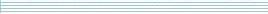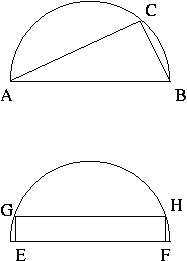| home      | people     | research     | publications    | seminars    | events     | contactCommunication Networks   | Systems Biology   | Hybrid Systems    | Machine Learning   | Dynamics & Interaction### Schools Mathematics Grand Challenge

Week seven's Puzzles

There are 3 problems this week. Don't worry if you find some of them a bit more tricky. If you get stuck, try looking at problem in a different way. If you don't think you'll be able to get the exact answer, you can always make an educated guess!

Problem 13:

Take a 2 digit number `ab` (so a number between 10 and 99). Form a 10 digit number from it by placing five copies of the number next to each other. For example, if the original number is 13, we form the 10 digit number

1313131313

or if the original number is 37 we form

3737373737.

There is exactly one 2 digit number such that when you add up the digits of the ten digit number obtained in this way, the sum is equal to the original number. What is it?

Problem 14:

A googol is 1 followed by 100 zeros, or 10^100.

If you check, you'll find that N=5 is the smallest number so that N! (N factorial) is divisible by 10. What is the smallest N so that N! is divisible by a googol?

Problem 15:

You inherit a 4 hectare semicircular plot of land in the centre of Dublin from an eccentric relative. A property developer offers to buy some land from you, however they are only interested in either triangular or rectangular plots of land. They will pay you 250,000 euro per hectare of land.Look at the two plots of land above. Your first option is to sell the triangle of land ABC. AB is the diameter of the semicircle and you can put C anywhere on the perimeter of the semicircle.

The second option is to sell a rectangle of land EFHG. You can put E and F anywhere on the base of the semicircle and G and H anywhere on the perimeter as long as EFHG is a rectangle.

What is the ratio of the value of the biggest triangular plot compared to the value of the biggest rectangular plot? Give your answer to 3 decimal places.

Hint 1: Remember your formulas for area.

Hint 2: On page 9 of the tables book you can find the formula: 2 cos(A) sin(A) = sin(2A). This means that the sine of twice an angle is equal to 2 times the product of the sine and cosine of the original angle.